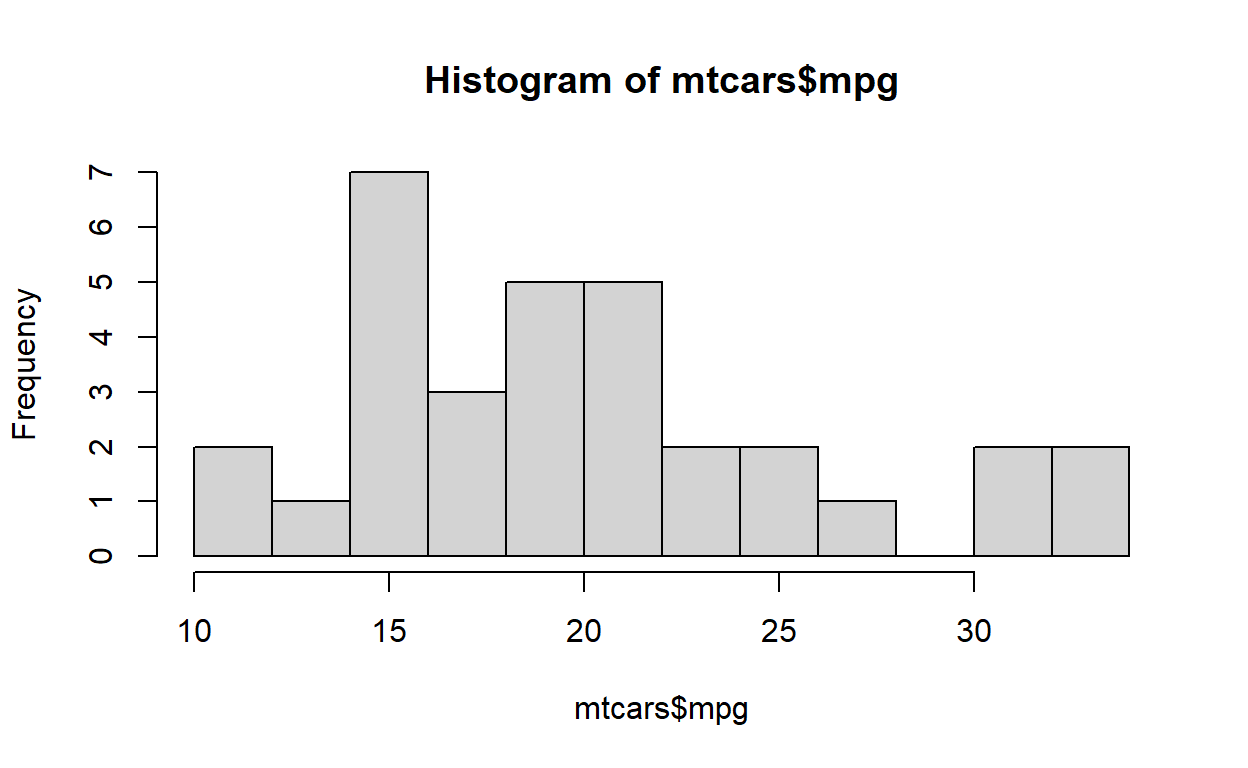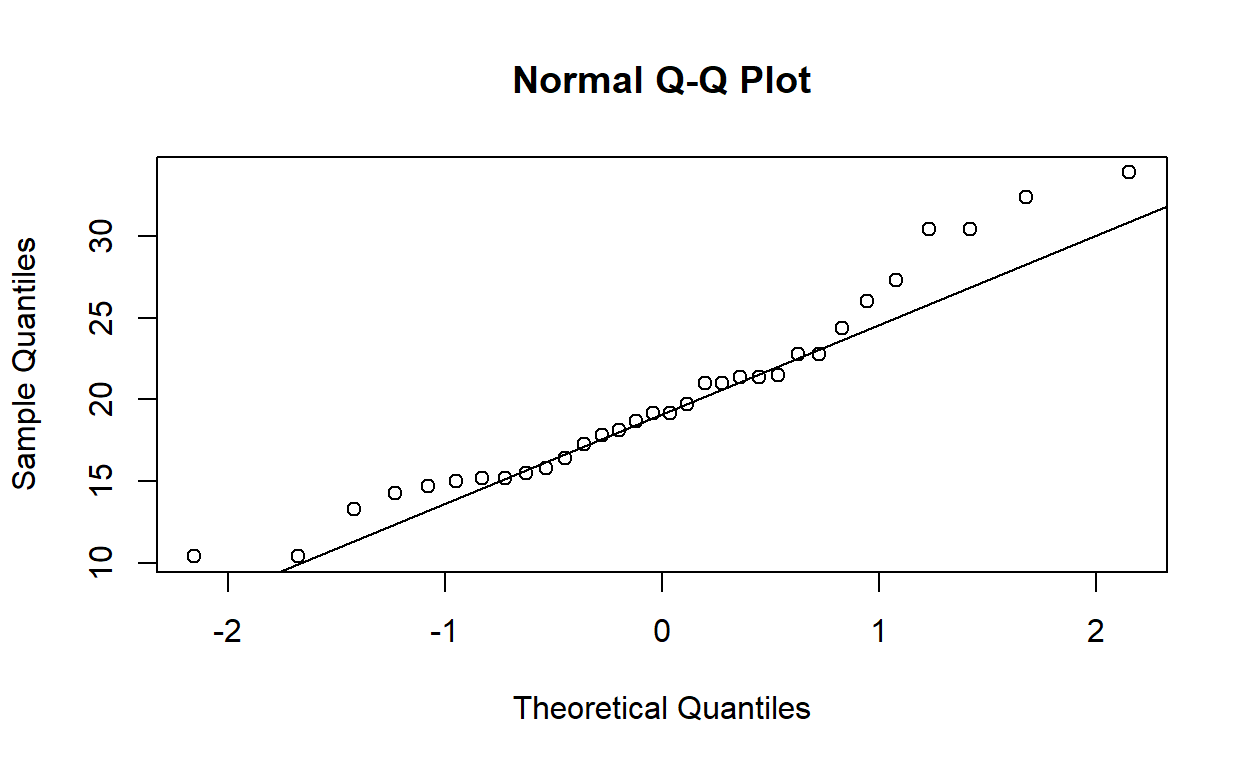# Statistics with R

Using R to to do statistics

Diwash Shrestha https://diwashrestha.rbind.io/rstats-1
04-20-2019

In this post we will discuss about the statistics with R.

### Introduction

Statistics is a branch of mathematics working with data collection, organization, analysis, interpretation and presentation.Statistics is very important in Data Analysis ,Data Science and AI.

In this post we will learn about the descriptive statistics with R.

### Descriptive Statistics

Descriptive Statistics is used to summarize the data and it focuses on Distribution , the central tendancy and dispersion of the data . In this section we will learn to work on

• Distribution
• Central tendency
• Dispersion

### Measures for central tendency

Central tendency is a measure that best summarizes the data and is a measure that is related to the center of the data set.Mean, median, and mode are the most common measures for central tendency.

We will use mtcars dataset from the datasets package in R.

head(mtcars)
                   mpg cyl disp  hp drat    wt  qsec vs am gear carb
Mazda RX4         21.0   6  160 110 3.90 2.620 16.46  0  1    4    4
Mazda RX4 Wag     21.0   6  160 110 3.90 2.875 17.02  0  1    4    4
Datsun 710        22.8   4  108  93 3.85 2.320 18.61  1  1    4    1
Hornet 4 Drive    21.4   6  258 110 3.08 3.215 19.44  1  0    3    1
Hornet Sportabout 18.7   8  360 175 3.15 3.440 17.02  0  0    3    2
Valiant           18.1   6  225 105 2.76 3.460 20.22  1  0    3    1

### Mean

The mean is the average of the data. It is the sum of all data divided by the number of data points. mean() function gives the mean of the data.

## mean of the mpg column
mean(mtcars$mpg)  20.09062 ### Median The median is the Middle or midpoint of the data and is also the 50 percentile of the data. The median is not affected by the outliers and skewness of the data. Median() function is used to get Median. ## median of the cyl median(mtcars$cyl)
 6

### Mode

Mode is a value in data that has highest frequency and useful when the differences are non-numeric and seldom occur.

 y <- table(mtcars$gear) names(y)[which(y ==max(y))]  "3" “3” is the mode of the gear column. ### Measures of variability Measure of variability are the measures of the spread of the data. It can be range ,interquartile range, variance, standard deviation. ### Range Range is the difference between the largest and smallest points in the data. range() function is used to find the range in R. range(mtcars$disp)
  71.1 472.0

### Interquartile Range

The interquartile range is the measure of the difference between the 75 percentile or third quartile and the 25 percentile or first quartile. IQR() function is used to get interquartile Range in R.

## IQR of sugar
IQR(mtcars$wt)  1.02875 quantile() function is used to get quartiles in R. ## quartile of the sugar quantile(mtcars$am)
  0%  25%  50%  75% 100%
0    0    0    1    1 

We can get the 25 and 75 percentiles of sugar in data.

quantile(mtcars$mpg, 0.25)  25% 15.425  quantile(mtcars$mpg, 0.75)
 75%
22.8 

### Variance

The variance is the average of squared differences from the mean and it is used to measure the spreadness of the data. var() function is used to find the sample variance in R.

var(mtcars$mpg)  36.3241 var() and (N-1)/N is used to find the population variance. N <- nrow(mtcars) var(mtcars$mpg) * (N -1) / N
 35.18897

### Standard Deviation

The standard deviation is the square root of a variance and it measures the spread of the data.

sd() is used to find the sample standard deviation of a dataset.

## standard deviation of the calcium
sd(mtcars$mpg)  6.026948 ### Normal Distribution Normal distribution is one of the most important theories because nearly all statistical tests require the data to be distributed normally. We can plot a distribution in R using hist() function. hist(mtcars$mpg, breaks = 15)qqnorm() and qqline functions are used to find whether data is normally distributed.

qqnorm(mtcars$mpg) qqline(mtcars$mpg)If the points do not deviate away from the line , the data is normally distributed.

### Modality

The modality of a distribution is determined by the number of peaks it contains.

hist(mtcars$mpg, breaks = 15)### Skewness and Kurtosis Skewness is a measurement of the symmetry of a distribution and how much the distribution is different from the normal distribution. Negative Skew is alos known as left skewed and positive skew is also known as right skewed. Th histogram from the previous section has a positive skew. Kurtosis measures whether your dataset is heavy-tailed or light-tailed compared to a normal distribution. High Kurtosis means heavy tailed , so there are more outliers in the data. To find the kurtosis and skewness in R , we need moments package. library(moments) ## skewness of the total_fat skewness(mtcars$mpg)

## Kurtosis of the total fat
kurtosis(mtcars$mpg)  0.6404399  2.799467 ### summary() and str() function The summary() and str() function are the fastest ways to get descriptive statistics of the data. We can get the basic descriptive statistics using the summary() function. summary(mtcars)  mpg cyl disp hp Min. :10.40 Min. :4.000 Min. : 71.1 Min. : 52.0 1st Qu.:15.43 1st Qu.:4.000 1st Qu.:120.8 1st Qu.: 96.5 Median :19.20 Median :6.000 Median :196.3 Median :123.0 Mean :20.09 Mean :6.188 Mean :230.7 Mean :146.7 3rd Qu.:22.80 3rd Qu.:8.000 3rd Qu.:326.0 3rd Qu.:180.0 Max. :33.90 Max. :8.000 Max. :472.0 Max. :335.0 drat wt qsec vs Min. :2.760 Min. :1.513 Min. :14.50 Min. :0.0000 1st Qu.:3.080 1st Qu.:2.581 1st Qu.:16.89 1st Qu.:0.0000 Median :3.695 Median :3.325 Median :17.71 Median :0.0000 Mean :3.597 Mean :3.217 Mean :17.85 Mean :0.4375 3rd Qu.:3.920 3rd Qu.:3.610 3rd Qu.:18.90 3rd Qu.:1.0000 Max. :4.930 Max. :5.424 Max. :22.90 Max. :1.0000 am gear carb Min. :0.0000 Min. :3.000 Min. :1.000 1st Qu.:0.0000 1st Qu.:3.000 1st Qu.:2.000 Median :0.0000 Median :4.000 Median :2.000 Mean :0.4062 Mean :3.688 Mean :2.812 3rd Qu.:1.0000 3rd Qu.:4.000 3rd Qu.:4.000 Max. :1.0000 Max. :5.000 Max. :8.000  We can get the structure of the data using the str() function. str(mtcars) 'data.frame': 32 obs. of 11 variables:$ mpg : num  21 21 22.8 21.4 18.7 18.1 14.3 24.4 22.8 19.2 ...
$cyl : num 6 6 4 6 8 6 8 4 4 6 ...$ disp: num  160 160 108 258 360 ...
$hp : num 110 110 93 110 175 105 245 62 95 123 ...$ drat: num  3.9 3.9 3.85 3.08 3.15 2.76 3.21 3.69 3.92 3.92 ...
$wt : num 2.62 2.88 2.32 3.21 3.44 ...$ qsec: num  16.5 17 18.6 19.4 17 ...
$vs : num 0 0 1 1 0 1 0 1 1 1 ...$ am  : num  1 1 1 0 0 0 0 0 0 0 ...
$gear: num 4 4 4 3 3 3 3 4 4 4 ...$ carb: num  4 4 1 1 2 1 4 2 2 4 ...

In this blog i wrote about the basic or descriptive statistics with R. In another blog i will write about the inferential part of statistics which is very important used mostly in research and analysis.